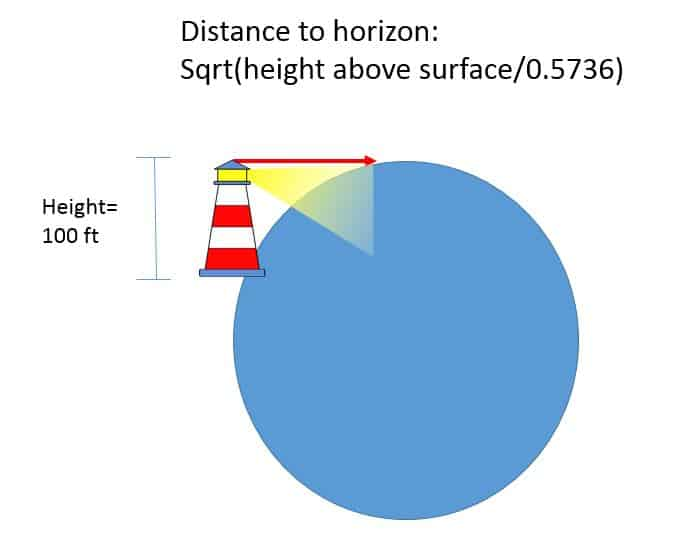# How to calculate distance to horizon

Imagine you’re on a boat in the ocean and in the far distance a sliver of land appears. You wonder: How can we calculate that distance between us and that piece of land. The distance depends on two things: the curvature of the earth (a known entity) and the height of your eyes from the water’s surface.

We can use height above the surface in feet and distance to horizon in miles. Let’s use the equation: sqrt(height above surface/0.5736)= distance to the horizon.

Let’s look at a 100 ft tall light house and calculate the distance the light shines to the horizon (assuming the light travels straight.we’ll plug in 100′ to the formula, so now we have sqrt(100/.5736)= 13.2 miles. Hopefully that visability is enough for passing ships.